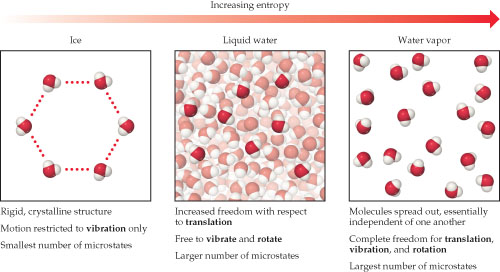# Problem: Entropy and the phases of water. The larger the number of possible microstates, the higher the entropy of the system.In which phase are water molecules least able to have rotational motion?

###### FREE Expert Solution

We’re asked to determine which phase are water molecules least able to have rotational motion given the diagram showing how entropy increases as water changes from ice to liquid and liquid to water vapor.

Recall that entropy is the degree of chaos or disorder of a system. Based on the diagram, the larger the number of possible microstates, the higher the entropy of the system.

Rotational motion involves an entire molecule rotating or the internal parts of the molecule rotating with respect to each other.

As can bee seen on the diagram:

96% (292 ratings)###### Problem DetailsEntropy and the phases of water. The larger the number of possible microstates, the higher the entropy of the system.

In which phase are water molecules least able to have rotational motion?